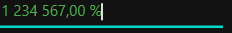# Getting Started with WPF Percent TextBox

30 Jan 20239 minutes to read

This section explains how to create a WPF `PercentTextBox` control and its features.

## Assembly deployment

Refer to the control dependencies section to get the list of assemblies or NuGet package that needs to be added as a reference to use the control in any application.

You can find more details about installing the NuGet package in a WPF application in the following link:

How to install nuget packages

## Adding WPF PercentTextBox via designer

You can add the PercentTextBox control to an application by dragging it from the toolbox to a view of the designer. The following dependent assembly will be added automatically:

• Syncfusion.Shared.WPF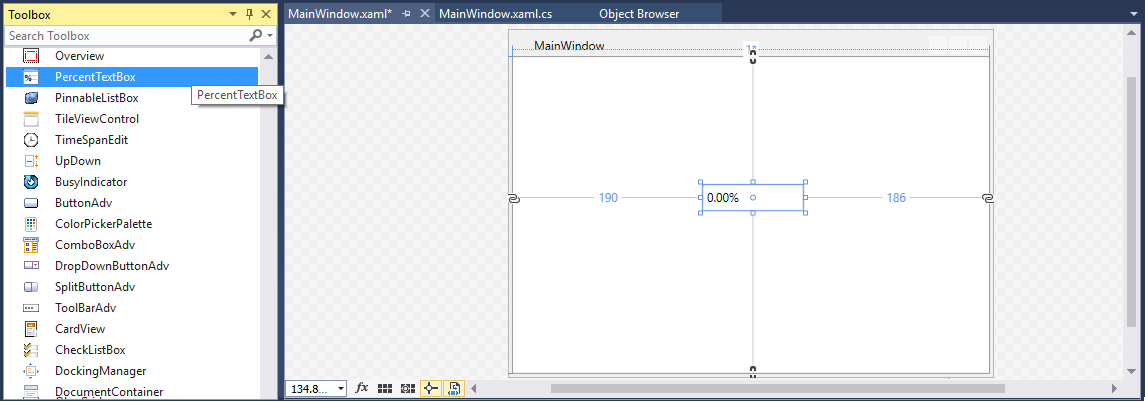## Adding WPF PercentTextBox via XAML

1. Create a new WPF project in Visual Studio.

2. Add the Syncfusion.Shared.WPF assembly references to the project.

3. Import Syncfusion WPF schema http://schemas.syncfusion.com/wpf and declare the `PercentTextBox` control in XAML page.

``````<Window xmlns="http://schemas.microsoft.com/winfx/2006/xaml/presentation"
xmlns:x="http://schemas.microsoft.com/winfx/2006/xaml"
xmlns:syncfusion="http://schemas.syncfusion.com/wpf"
x:Class="PercentTextBoxSample.MainWindow"
Title="PercentTextBox Sample" Height="350" Width="525">
<Grid>
<syncfusion:PercentTextBox x:Name="percentTextBox" Width="100" Height="25" VerticalAlignment="Center" HorizontalAlignment="Center"/>
</Grid>
</Window>``````

## Adding WPF PercentTextBox via C#

1. Create a new WPF application via Visual Studio.

2. Add the Syncfusion.Shared.WPF assembly references to the project.

3. Include the required namespace.

``using Syncfusion.Windows.Shared;``
4. Create an instance of PercentTextBox and add it to the window.

``````//Creating an instance of PercentTextBox control

PercentTextBox percentTextBox = new PercentTextBox();

// Setting height and width to PercentTextBox

percentTextBox.Height = 25;
percentTextBox.Width = 100;

this.Content = percentTextBox;``````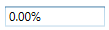## Setting Value

The percent value of the `PercentTextBox` can be set by using the PercentValue property.

``<syncfusion:PercentTextBox x:Name="percentTextBox" Width="100" Height="23" PercentValue="100"/>``
``````PercentTextBox percentTextBox = new PercentTextBox();
percentTextBox.Width = 100;
percentTextBox.Height = 23;
percentTextBox.PercentValue = 100;``````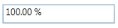NOTE

Do not use the Text property to set the percent value for the PercentTextBox. Use only the `PercentValue` property.

### Binding Value

Data binding is the method of forming a connection between the application UI and business logic. Data binding can be unidirectional (source -> target or target <- source) or bidirectional (source <-> target). You can bind data to the `PercentTextBox` using the `Value` Property.

The following code snippets illustrate the percent value binding from one `PercentTextBox` to another.

``````<StackPanel>
<syncfusion:PercentTextBox x:Name="percentTextBox1" Height="25" Width="100" PercentValue="{Binding MyValue,UpdateSourceTrigger=PropertyChanged}"/>
<syncfusion:PercentTextBox x:Name="percentTextBox2" Width="100" Height="25" PercentValue="{Binding MyValue,UpdateSourceTrigger=PropertyChanged}" />
</StackPanel>``````

ViewModel.cs

``````class ViewModel : NotificationObject
{
private double myValue;
public double MyValue
{
get
{
return myValue;
}
set
{
myValue = value;
RaisePropertyChanged("MyValue");
}
}
}``````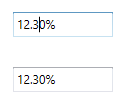The `PercentTextBox` control can notifies the percent value changes through the PercentValueChanged event. You can get old percent value and new percent value from `OldValue` and `NewValue` properties in `PercentValueChanged` event.

``<syncfusion:PercentTextBox PercentValueChanged="PercentTextBox_PercentValueChanged"/>``
``````PercentTextBox percentTextBox = new PercentTextBox();
percentTextBox.PercentValueChanged += new PropertyChangedCallback(PercentTextBox_PercentValueChanged);``````

You can handle the event as follows:

``````private void PercentTextBox_PercentValueChanged(DependencyObject d, DependencyPropertyChangedEventArgs e)
{
// Get old and new percent value
var newValue = e.NewValue;
var oldValue = e.OldValue;
}``````

## Min Max Value Restriction

The `PercentValue` of `PercentTextBox` can be restricted within maximum and minimum limit. You can define the minimum and maximum values by setting the MinValue and MaxValue properties. It allows the user to enter the percent value between `MinValue` and `MaxValue`.

``<syncfusion:PercentTextBox x:Name="percentTextBox" Width="100" Height="25" PercentValue="100" MaxValue="999.99" MinValue="-999.99"/>``
``````PercentTextBox percentTextBox = new PercentTextBox();
percentTextBox.Width = 100;
percentTextBox.Height = 25;
//Setting minimum value
percentTextBox.MinValue = -999.99;
//Setting maximum value
percentTextBox.MaxValue = 999.99;
percentTextBox.PercentValue = 100;``````## Step Interval to increase or decrease the value

The `PercentTextBox` control allows to increase or decrease the percent value by pressing up and down arrow keys in keyboard or mouse wheel over the control. The ScrollInterval property is used to specify the increment or decrement intervals. The default value of `ScrollInterval` is 1.

``````<syncfusion:PercentTextBox x:Name="percentTextBox" Width="150" Height="25" PercentValue="8"
IsScrollingOnCircle="True" ScrollInterval="4"/>``````
``````PercentTextBox percentTextBox = new PercentTextBox();
percentTextBox.Width = 150;
percentTextBox.Height = 25;
percentTextBox.MinValue = 0;
percentTextBox.MaxValue = 100;
percentTextBox.PercentValue = 8;
percentTextBox.IsScrollingOnCircle = true;
percentTextBox.ScrollInterval = 4;``````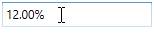## Formatting the value

You can customize the number format by either setting the NumberFormat property or the PercentGroupSeparator, PercentGroupSizes, PercentDecimalDigits, PercentDecimalSeparator, PercentNegativePattern, PercentPositivePattern, and PercentageSymbol properties of `PercentTextBox`.

``<syncfusion:PercentTextBox x:Name="percentTextBox" Height="25" Width="150" PercentValue="1234567" PercentageSymbol="%" PercentDecimalDigits="4" PercentDecimalSeparator="*" PercentGroupSeparator="/"/>``
``````PercentTextBox percentTextBox = new PercentTextBox();
percentTextBox.Width = 150;
percentTextBox.Height = 25;
percentTextBox.PercentValue = 1234567;
percentTextBox.PercentageSymbol = "%";
percentTextBox.PercentDecimalDigits = 4;
percentTextBox.PercentDecimalSeparator = "/";
percentTextBox.PercentGroupSeparator = "*";``````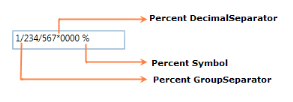## Setting the Culture

The `PercentTextBox` provides support for globalization by using the Culture property. The `Culture` is used to format the decimal separator and group separator of the `PercentTextBox` percent value based on the respective culture.

``<syncfusion:PercentTextBox x:Name="percentTextBox" Height="25" Width="150" Culture="en-US" PercentValue="1234567"/>``
``````PercentTextBox percentTextBox = new PercentTextBox();
percentTextBox.Width = 150;
percentTextBox.Height = 25;
percentTextBox.PercentValue = 1234567;
percentTextBox.Culture = new System.Globalization.CultureInfo("en-US");``````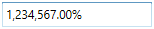NOTE

When you use both `NumberFormat` and `Culture`, the `NumberFormat` will have a higher priority.

## Theme

PercentTextBox supports various built-in themes. Refer to the below links to apply themes for the PercentTextBox,